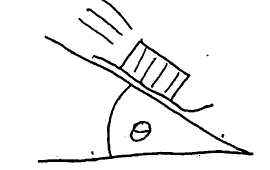# Problem: A box on sled slides down a long, snow-covered slope. The hill slopes at a constant angle θ. There is a small coefficient of kinetic friction of 0.19. Find an expression for the acceleration.

###### FREE Expert Solution
96% (165 ratings)
###### Problem Details

A box on sled slides down a long, snow-covered slope. The hill slopes at a constant angle θ. There is a small coefficient of kinetic friction of 0.19. Find an expression for the acceleration.